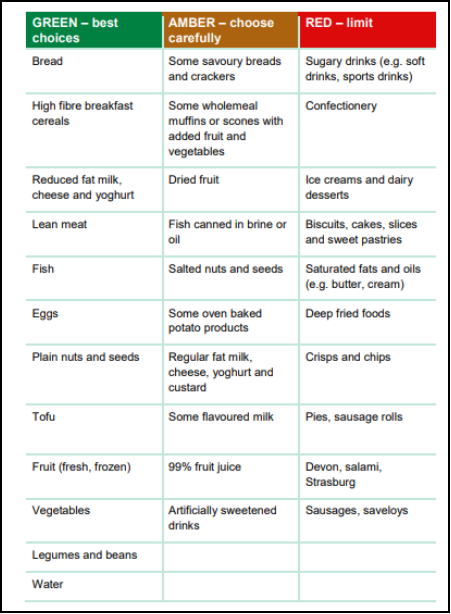# Supply And Demand For SkateboardsPages: 4Words: 890

## Question :

Hand-Up 2 (Week 5)

Instructions

• This hand-up is based on Topics 2 and 3 of BUS114.
• You must submit this hand-up at the beginning of class in Week 5. The hand-up will be returned to you with feedback in Week 6.
• The hand-up must be hand written.
• Your best 3 out of 4 hand-ups count for 25% of your final mark.
• Submissions which are 1) through email 2) typed or 3) handed in late will not be accepted.

Consider the following supply and demand schedules for skateboards.

 Price (\$) Quantity Demanded (thousands per month) Quantity Supplied (thousands per month) 550 60 130 500 65 120 450 70 110 400 75 95 350 80 80 300 85 75 250 90 70 200 95 65

Question 1

State the market equilibrium price and quantity of skateboards. (1 mark)

Question 2

Sketch the market for skateboards in a diagram, making sure to include all appropriate labels. (1 mark)

Question 3

If the supplier of the skateboards decided to restrict the price of a skateboard to be \$500, would this result in a shortage or a surplus? Explain why. What is the size of the surplus or shortage? (2 marks)

Question 4

Say Donald Trump was photographed riding one of these skateboards outside the White House. What effect might this have on the demand for these skateboards, and which way would the demand curve shift? (1 mark)

Q.1.

Ans-

 Price Quantity Demanded Quantity Supplied 550 60 130 500 65 120 450 70 110 400 75 95 350 80 80 300 85 75 250 90 70 200 95 65

At equilibrium, quantity demanded is equal to quantity supplied.

Qd = Qs

Let us suppose the following to be the demand and supply function respectively-

Qd = a – bP

= 115-0.1P

Qs = a + bP

= 20+0.2P

Hence, equilibrium-

Qd = Qs

115-0.1P = 20+0.2P

0.3P =95

P = 32

Equilibrium demand-

Qd= 115 – 0.1 (32) = 112 [approx.]

Qs= 20 + 0.2 (32) = 26 [approx.]

Q.2.

Ans- Following is the diagramThe enclosed area represented by Op*q* is the market for skateboards.

Q.3.

Ans-

Qs= 20+0.2P

= 20 + 0.2 

= 120

Qd = 115-0.1P

= 115 – 0.1 

= 15

With price being fixed at \$500, the price is on the higher side of the chart, so the demand will fall and the supply will be in excess. Therefore, it is a case of surpus.

Q.4.

Ans- The demand curve would shift to the right.

Right shift in a demand curve is indicative of increase in quantity demanded because of other than price factors. In this case, Donald Trump is a celebrity and if he will be photographed riding one of these skateboards outside the White House, the demand for the skateboard will increase. Economics therefore functions in this particular way.

Tags: